# Binary Search Tree - Delete Operation in C++

Binary search tree (BST) is a special type of tree which follows the following rules −

• left child node’s value is always less than the parent Note

• right child node has a greater value than the parent node.

• all the nodes individually form a binary search tree.

Example of a binary search tree (BST)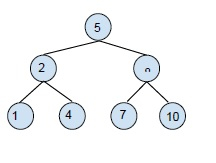A binary search tree is created in order to reduce the complexity of operations like search, find minimum and maximum.

## Delete Operation binary search tree (BST)

delete operation is dropping the specified node from the tree. in case deleting the nodes, there are three possibilities −

• Deleting a leaf node from the tree: The simplest deletion is the deletion of a leaf node from the binary search tree. For deleting the leaf node only the leaf gets affected. Example,deleting the leaf node 7 gives,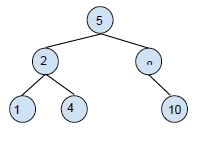• Deleting the node with one child node: for this deletion, you need to replace the child node with the node to be deleted and then delete it. Example,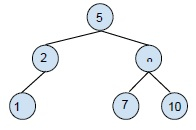Deleting 2 from the BST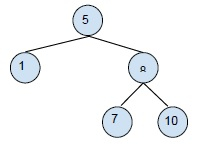• Deleting the node with two child nodes: Here the node to be deleted has two child nodes. So, we will use in the order form of the tree, here we will delete the element and select its inorder neighbor for its place and recreate the rest. Example,Deleting 5 from the BST will return the following tree.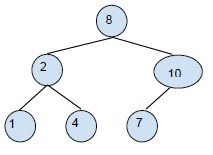## Example

Live Demo

#include<stdio.h>
#include<stdlib.h>
struct node{
int key;
struct node *left, *right;
};
struct node *newNode(int item){
struct node *temp = (struct node *)malloc(sizeof(struct node));
temp->key = item;
temp->left = temp->right = NULL;
return temp;
}
void inordertraversal(struct node *root){
if (root != NULL){
inordertraversal(root->left);
printf("%d ", root->key);
inordertraversal(root->right);
}
}
struct node* insert(struct node* node, int key){
if (node == NULL) return newNode(key);
if (key < node->key)
node->left = insert(node->left, key);
else
node->right = insert(node->right, key);
return node;
}
struct node * minValueNode(struct node* node){
struct node* current = node;
while (current && current->left != NULL)
current = current->left;
return current;
}
struct node* deleteNode(struct node* root, int key){
if (root == NULL) return root;
if (key < root->key)
root->left = deleteNode(root->left, key);
else if (key > root->key)
root->right = deleteNode(root->right, key);
else{
if (root->left == NULL){
struct node *temp = root->right;
free(root);
return temp;
}
else if (root->right == NULL){
struct node *temp = root->left;
free(root);
return temp;
}
struct node* temp = minValueNode(root->right);
root->key = temp->key;
root->right = deleteNode(root->right, temp->key);
}
return root;
}
int main(){
struct node *root = NULL;
root = insert(root, 50);
root = insert(root, 30);
root = insert(root, 20);
root = insert(root, 40);
root = insert(root, 70);
root = insert(root, 60);
root = insert(root, 80);
printf("Inorder traversal of the given tree \n");
inordertraversal(root);
printf("\nDelete 20\n");
root = deleteNode(root, 20);
printf("Inorder traversal of the modified tree \n");
inordertraversal(root);
printf("\nDelete 30\n");
root = deleteNode(root, 30);
printf("Inorder traversal of the modified tree \n");
inordertraversal(root);
printf("\nDelete 50\n");
root = deleteNode(root, 50);
printf("Inorder traversal of the modified tree \n");
inordertraversal(root);
return 0;
}

## Output

Inorder traversal of the given tree
20 30 40 50 60 70 80
Delete 20
Inorder traversal of the modified tree
30 40 50 60 70 80
Delete 30
Inorder traversal of the modified tree
40 50 60 70 80
Delete 50
Inorder traversal of the modified tree
40 60 70 80